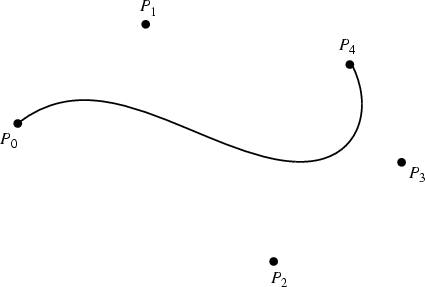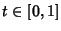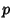## Bézier CurveGiven a set ofcontrol points, the corresponding Bézier curve (or Bernstein-Bézier Curve) is given bywhereis a Bernstein Polynomial and.

A rational'' Bézier curve is defined bywhereis the order,are the Bernstein Polynomials,are control points, and the weightofis the last ordinate of the homogeneous point. These curves are closed under perspective transformations, and can represent Conic Sections exactly.

The Bézier curve always passes through the first and last control points and lies within the Convex Hull of the control points. The curve is tangent toandat the endpoints. The variation diminishing property'' of these curves is that no line can have more intersections with a Bézier curve than with the curve obtained by joining consecutive points with straight line segments. A desirable property of these curves is that the curve can be translated and rotated by performing these operations on the control points.

Undesirable properties of Bézier curves are their numerical instability for large numbers of control points, and the fact that moving a single control point changes the global shape of the curve. The former is sometimes avoided by smoothly patching together low-order Bézier curves. A generalization of the Bézier curve is the B-Spline.